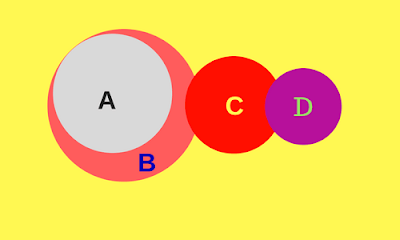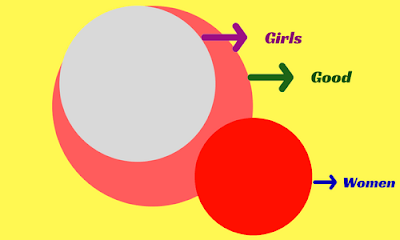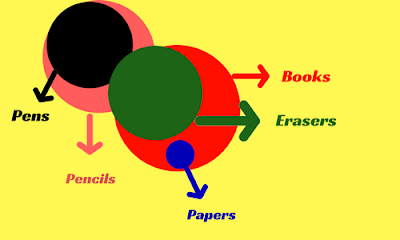New Student User - Use Code HELLO

# Syllogism Tricks Explained With ExamplesThis is one of the very important topics that is frequently asked in competitive exams. Theory on Syllogisms has been published on 10-Jan-2017.Here we will discuss some examples.

#### Example 1):-

Statements:-
1) All A are B
2) Some B are C
3) No B is D

Conclusion:-
1) No C is D
2) No A is D
3) Some C are not D
Solution:-Above diagram is the Block Diagram (BD) from the given statements.
Conclusion (I) is negative & is true.
Conclusion (II) is negative & is true.
Conclusion (III) is negative & is true.
To prove conclusion (I), “No C is D”, to be false, we have to prove that its complementary conclusion, “Some C are D” is true. Hence, the AD for this will be as shown below.The above diagram does not defy any of the given statements. Conclusion (I), “No C is D”, is false for this diagram, hence is not valid.
To negate conclusion (II), “No A is D”, & (III), “Some C are not D”, we have to prove that their respective complementary conclusions “some A are D” & “All C are D”, are true. This possible, only if ‘D’ encroaches into ‘B’. But this will violate statement (3). Hence, no diagram can be drawn to negate these two conclusions.
Hence, only conclusion (II) & (III) follows.
Thus, we are able to answer the question with only two diagrams.

•  From BD, we can verify the truthfulness of each statement & accordingly decide whether a conclusion follows the given statements or not.
•  In the case of a negative conclusion becoming true, we go for AD to prove the negative conclusion false. If such AD is possible, the negative statement does not follow, otherwise, it follows the given statement.

#### Example 2):-

Statements:-
1) All girls are good
2) Some good are women

Conclusions:-
(I). some women are girls
(II). No woman is a girl.

Solution:-
Clearly, the above two conclusions form a complementary pair.
Basic Diagram:-Conclusion (I) is affirmative & is false.
Conclusion (II) is negative & is true.
Now, we should draw an AD, which would make the conclusion (II) false i.e., which proves “Some women are girls”.
Alternate Diagram:-From the above diagram, conclusion (II) is false, but conclusion (I) is true at the same time. But they both cannot be true or false at the same time. Hence, either (I) or (II) follows.

#### Example 3):-

Statements:-
1) All pens are pencils
2) Some pens are erasers
3) All papers are books
4) All erasers are books
Conclusions:-
(I) some pencils are books.
(II) some books are pens.
(III) No paper is pen.
(IV) some erasers are papers.
Solutions:-
The given statements can be represented in the following basic diagram.
Basic Diagram:-From the above diagram,
Conclusion (I), affirmative, follows.
Conclusion (II), affirmative, follows.
Conclusion (III), negative follows.
Conclusion (IV), affirmative, does not follow.
As the affirmative conclusions (I & II) are true in the BD, they will always be true. The affirmative conclusion (IV) is false in the basic diagram. Even if it is true in other diagrams, it cannot be said to be true as there is a situation, where it is false.
The negative conclusion (III), which is true in the basic diagram, has to be checked whether it can be false in any alternate diagram. The following is such diagram.
Alternate Diagram:-There is a situation, where conclusion (III) is false. Hence, only (I) & (II) are true & follows.